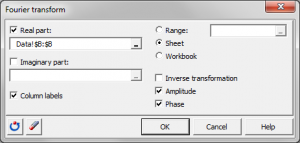# Fourier transform

Fourier transform is a popular tool that investigates frequencies that occur in a function. Run Fourier transforms in Excel with the XLSTAT software.## What is Fourier transformation

Fourier transformation transforms one complex-valued function of a real variable into another. The domain of the original function is typically time. The domain of the new function is typically called the frequency domain. It describes which frequencies are present in the original function. Technically, the Fourier transform decomposes a function into oscillatory functions. The expression Fourier transform refers both to the frequency domain representation of a function, and to the process or formula that transforms one function into the other.

## Where should we use Fourier transformation

Fourier transformation is used to transform a time series or a signal to its Fourier coordinates, or to do the inverse transformation. While the Excel function is limited to powers of two for the length of the time series, XLSTAT is not restricted. Outputs optionally include the amplitude and the phase.### analyze your data with xlstat

14-day free trial

Included in

Related features